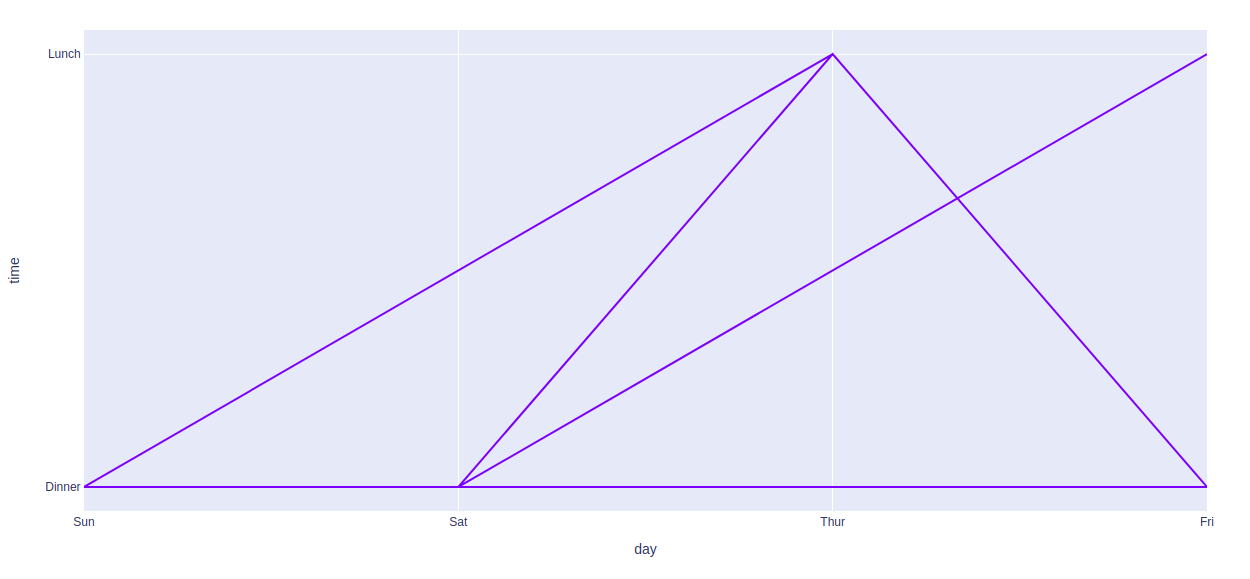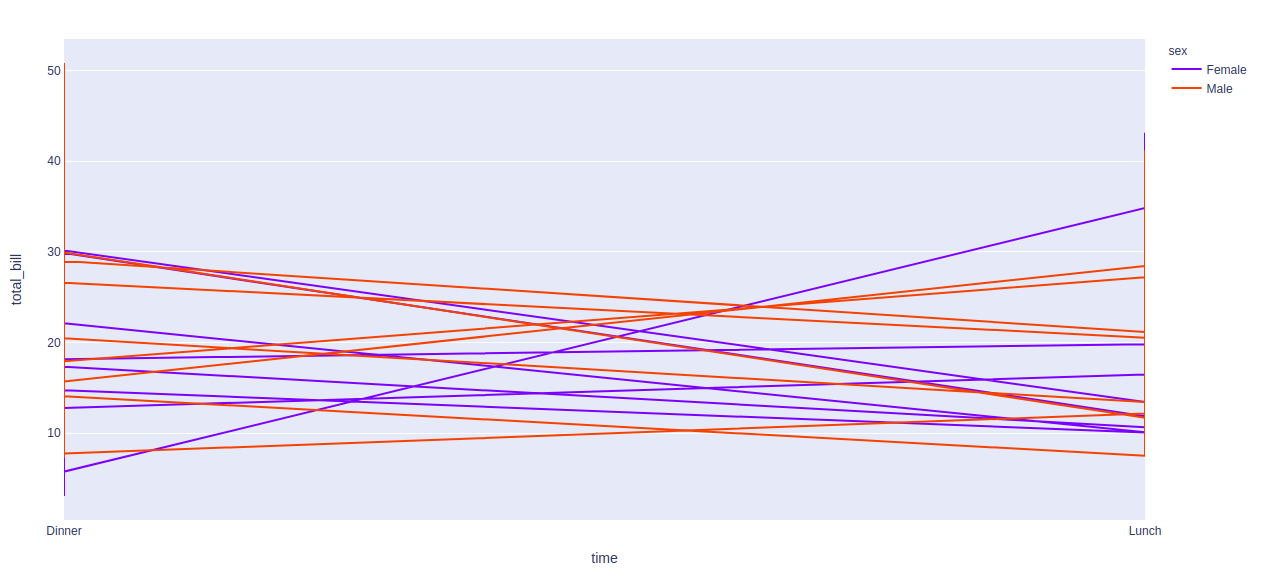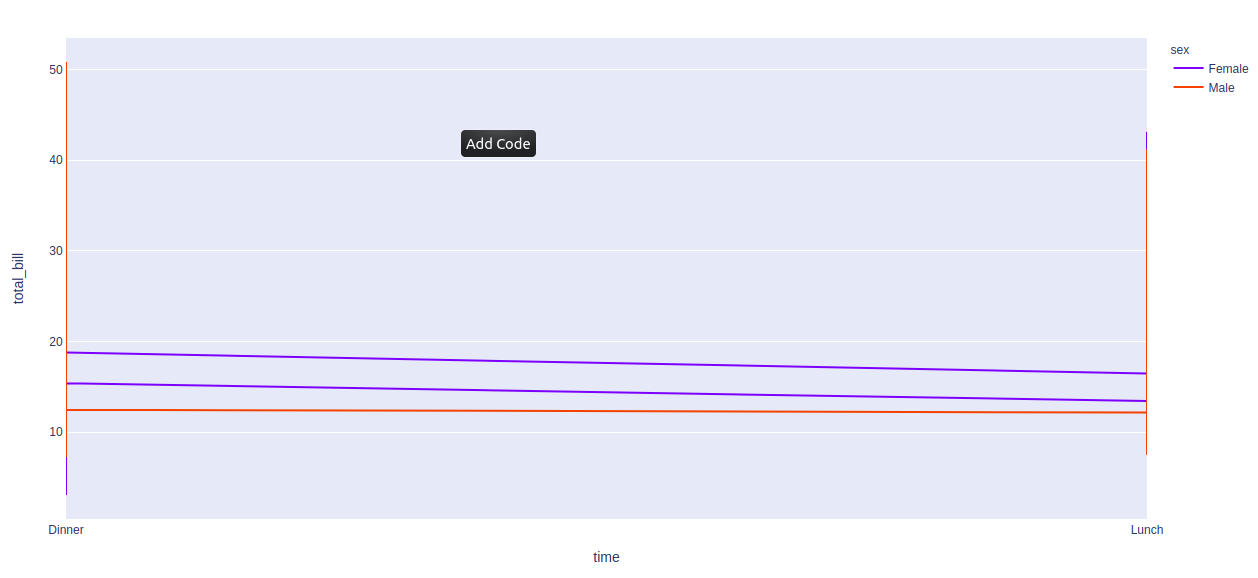Skip to content
Related Articles
plotly.express.line() function in Python
• Last Updated : 20 Jul, 2020

Plotly library of Python can be very useful for data visualization and understanding the data simply and easily. Plotly graph objects are a high-level interface to plotly which are easy to use.

## plotly.express.line() function

This function is used to create a line plot. It can also be created using the pandas dataframe where each row of data_frame is represented as vertex of a polyline mark in 2D space.

Syntax: plotly.express.line(data_frame=None, x=None, y=None, line_group=None, color=None, line_dash=None, hover_name=None, hover_data=None, title=None, template=None, width=None, height=None)

Parameters:

data_frame: DataFrame or array-like or dict needs to be passed for column names.

x, y: This parameters is either a name of a column in data_frame, or a pandas Series or array_like object. Values from this column or array_like are used to position marks along the x and y axis in cartesian coordinates respectively.

color: This parameters assign color to marks.

line_group: This parameter is used to group rows of data_frame into lines.

line_dash: This parameter is used to assign dash-patterns to lines.

hover_name:  Values from this column or array_like appear in bold in the hover tooltip.

hover_data: This parameter is used to appear in the hover tooltip or tuples with a bool or formatting string as first element, and list-like data to appear in hover as second element Values from these columns appear as extra data in the hover tooltip.

Example 1:

## Python3

 `import` `plotly.express as px`` ` `df ``=` `px.data.tips()`` ` `plot ``=` `px.line(df, x ``=` `'day'``, y ``=` `'time'``)``plot.show()`

Output:Example 2: Using color argument

## Python3

 `import` `plotly.express as px`` ` `df ``=` `px.data.tips()`` ` `plot ``=` `px.line(df, x ``=` `'time'``, ``               ``y ``=` `'total_bill'``,``               ``color ``=` `'sex'``)``plot.show()`

Output:Example 3: Using the line_group argument

## Python3

 `import` `plotly.express as px`` ` `df ``=` `px.data.tips()`` ` `plot ``=` `px.line(df, x ``=` `'time'``,``               ``y ``=` `'total_bill'``,``               ``color ``=` `'sex'``, ``               ``line_group ``=` `'day'``)`` ` `plot.show()`

Output:Attention geek! Strengthen your foundations with the Python Programming Foundation Course and learn the basics.

To begin with, your interview preparations Enhance your Data Structures concepts with the Python DS Course. And to begin with your Machine Learning Journey, join the Machine Learning – Basic Level Course

My Personal Notes arrow_drop_up